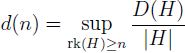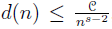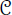# On subsets of finite Abelian groups without non-trivial solutions of x1 + x2 + … + xs – sxs+1 = 0

Ran Ji and Craig V. Spencer
Notes on Number Theory and Discrete Mathematics, ISSN 1310-5132
Volume 19, 2013, Number 3, Pages 55—59

## Details

### Authors and affiliations

Ran JiDepartment of Mathematics, Wellesley College
106 Central Street, Wellesley, MA 02481, USA

Craig V. SpencerDepartment of Mathematics, Kansas State University
138 Cardwell Hall, Manhattan, KS 66506, USA

### Abstract

Let D(G) be the maximal cardinality of a set AG that contains no non-trivial solution to x1 + … + xssxs+1 = 0 with xiA (1 ≤ is + 1). Letwhere rk(H) is the rank of H. We prove that for any n ∈ ℕ,, whereis a fixed constant depending only on s.

### Keywords

• Finite Abelian groups
• Character sums

• 11B30
• 20D60
• 11T24

### References

1. Lev, V. F. Progression-free sets in finite abelian groups, J. Number Theory Vol. 104, 2004, 162–169.
2. Liu, Y.-R., C. V. Spencer, A generalization of Meshulam’s Theorem on subsets of finite abelian groups with no 3-term arithmetic progression, Design. Code. Cryptogr., Vol. 52, 2009, 83–91.
3. Meshulam, R. On subsets of finite abelian groups with no 3-term arithmetic progressions, J. Combin. Theory Ser. A, Vol. 71, 1995, 168–172.
4. Serre, J.-P. A Course in Arithmetic, Springer-Verlag, New York, 1973.

## Cite this paper

APA

Ji, R., & Spencer, C. V. (2013). On subsets of finite Abelian groups without non-trivial solutions of x1 + x2 + … + xs sxs+1 = 0. Notes on Number Theory and Discrete Mathematics, 19(3), 55-59.

Chicago

Ji, Ran, and Craig V. Spencer. “On Subsets of Finite Abelian Groups without Non-trivial Solutions of x1 + x2 + … + xs sxs+1 = 0.” Notes on Number Theory and Discrete Mathematics 19, no. 3 (2013): 55-59.

MLA

Ji, Ran, and Craig V. Spencer. “On Subsets of Finite Abelian Groups without Non-trivial Solutions of x1 + x2 + … + xs sxs+1 = 0.” Notes on Number Theory and Discrete Mathematics 19.3 (2013): 55-59. Print.